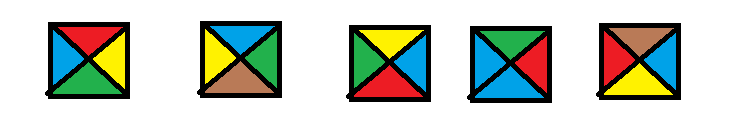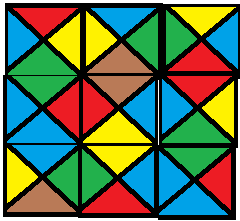# Monthly Archives: October 2019

## Crossword Puzzle Construction

Closing in on the end of the section, this is a “private communication” problem that I think I figured out myself.

The problem: Crossword Puzzle Construction.  This is problem GP14 in the appendix.

The description: Given a set W of words (strings over some finite alphabet Σ), and an n x n matrix A, where each element is 0 (or “clear”) or 1 (or “filled”).  Can we fill the 0’s of A with the words in W?  Words can be horizontal or vertical, and can cross just like crossword puzzles do, but each maximally contiguous horizontal or vertical segment of the puzzle has to form a word.

Example: Here’s a small grid.  *’s are 1, dashes are 0:

 * – – – * – – – – – – – – – – * * – – * * * * – *

Suppose our words were: {SEW, BEGIN, EAGLE, S, OLD, SEA, EGGO, WILLS, BE, SEA, NED}.  (Notice the lone “S” is a word.  That’s different from what you’d see in a normal crossword puzzle)

We can fill the puzzle as follows:

 * S E W * B E G I N E A G L E * * O L D * * * S *

Notice that we can’t use the first two letters of “BEGIN” as our “BE”, because the word continues along.  That’s what the “maximally contiguous” part of the definition is saying.

Reduction: From X3C. We’re given a set X with 3q elements, and a collection C of 3-element subsets of X.  We’re going to build a 3q x q puzzle with no black squares. (We’ll get back to making this a square in a minute)  Each word in W will be a bitvectorof length 3q, with a 0 in each position that does not have an element, and a 1 in the positions that do.  So, if X was {1,2,3,4,5,6,7,8,9} the set {1,3,5} would be 101010000

We also add to A the 3q bitvectors that have exactly one 1 (and 0’s everywhere else). The goal is to find a subset of C across the “rows” of the puzzle, such that the “columns” of the puzzle form one of the bitvectors.  If we can form each of the bitvectors, we have found a solution to X3C.  If we have a solution to X3C, we can use the elements in C’ and place them in the rows of the 3q x q puzzle block to come up with a legal crossword puzzle.

We’re left with 2 additional problems:  The grid needs to be a square, instead of a 3q x q rectangle, and the legal crossword puzzle solution needs to use all of the words in W, not the the ones that give us a C’.  We can solve both by padding the grid with blank squares.  Spaced out through the blank spaces are 1 x 3q sections of empty space surrounded by black squares.  We can put any word in C-C’ in any of these sections, and that’s where we’ll put the words that are not used.

(This also means we’ll have to add 3x|(C’-C)| 1’s and (3q-3)|(C’-C)| 0’s to our word list for all of the 1-length words in those  columns.)  Then we add enough blank spaces around the grid to make it a square.

Difficulty: 5 if I’m right, mainly because of the extra work you have to do at the end.  The comments in G&J say that the problem is NP-Complete “even if all entries in A are 0”, which is usually a hint that the “actual” reduction used an empty square grid.  I wonder if that reduction doesn’t have my hacky stuff at the end.

## Square Tiling

The reference in G&J is to an “unpublished result” (by Garey and Johnson themselves, with Papadimitriou).  I think the solution I found is not the one they are referring to.

The problem: Square Tiling.  This is problem GP13 in the appendix.

The description: Given a set C of colors, and a set T of tiles, where each of the 4 sides of the tile are colors from C (listed as a 4-tuple of (top, right, bottom, left) colors), and an integer N.  Can we tile an NxN square of tiles using tiles from C?  We can use a tile more than once, the tiles can’t be rotated, and adjacent edges need to match colors.

Example: Here are some example tiles I drew:We can tile these into a 3×3 grid like this:Reduction: As I said above, the reference in G&J is to an “unpublished result” by Garey, Johnson, and Papadimitriou.  I did manage to find a “generic reduction” using Turing Machines in the Lewis and Papadimitriou Theory book.

The reduction is from the “N2” language in the book, which (I think) is “Can a Turing Machine M halt in t steps or less with its head on the tape in configration uσv, where u and v are strings in Σ*, and σ is the location of the head (and a symbol in the alphabet)?

The idea is that we’ll build a set of tiles whose colors are based on the number of steps the computation has done so far.  The colors are actually tuples.  So, we have several kinds of tiles:

• For each a in Σ*, and each k from 1 to t, a tile with color (a, k+1) on the top, and (a,k) on the bottom.  This simulates a move that stays in the same state and writes the same symbol.
• For each pair a,b in Σ*, and each state p,q in M (p can include the halt state, q can’t), a tile with the color (p,b,k+1) on the top and (q,a,k) on the bottom.  This simulates going from state p to state q and replacing an a with a b.
• We can transition from one of the above types of tiles to another using a sideways move.  (If the head moves left or right, we move to a tile to the left or right)
• There are special tiles for the start row and for when the machine halts.

We set our N (the size of the tiling grid we’re making) to t+2.  What we’ve built is a system that:

• Has to have the tiles corresponding to one of the start states on the bottom row
• Has in row i a tile corresponding to a configuration of the machine after i steps.  (A path of tiles from the bottom to row i show the computation needed to get to that state)
• Has at the top row a tile corresponding to a configuration after t+1 steps.  If there is a legal tiling, one of those tiles must contain the halt state.

..which they claim is the same as the N2 langauge.

The suggested reduction in the appendix is from Hamiltonian Path, and I spent some time thinking about how to make that work, but couldn’t do it.  Do you make tiles correspond to vertices? Do you make colors correspond to vertices?  How do you account for the fact that you can go in two dimensions?  How do you account for the fact that you don’t know the order in which you visit the tiles?  I think it might be similar to this way of thinking about configurations.

Difficulty: 9 because it’s such a non-standard way of doing things.  I bet the Hamiltonian Path reduction is a lot easier.